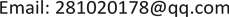1. 引言

2. 方法2.1. 被试

2.2. 实验设计

Characteristics of three groups of participant

ANOVA差异检验F(2,87) = 0.58, p = 0.56F(2,87) = 2.22, p = 0.114F(2,87) = 70.05, p = 0.000**F(2,87) = 24.95, p = 0.000**

2.3. 工具和材料

2.4. 程序

3. 结果3.1. 初步分析

3.2. 注意偏向

Description statistics of reaction time and accuracy of different subjects and matrix type

4. 讨论4.1. 对食物刺激的注意警觉

4.2. 对食物刺激的注意回避

5. 研究不足与展望

6. 结论